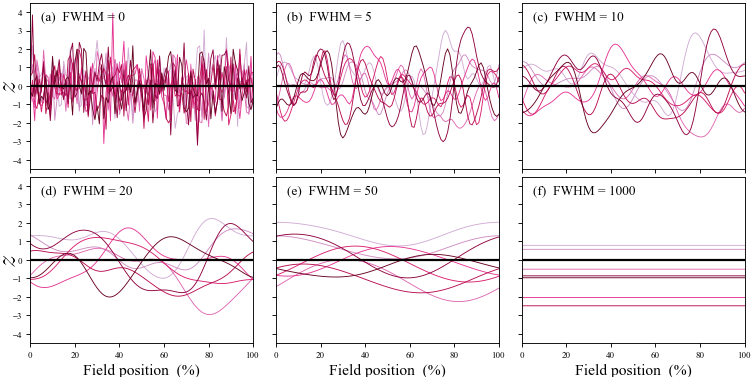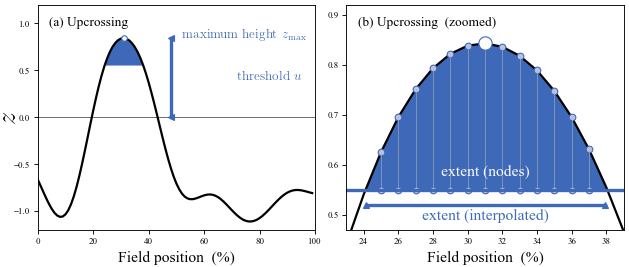# RFT Overview¶

RFT describes the probabilities that smooth, random fields will:

1. reach particular heights, and
2. produce upcrossings which extend beyond particular heights and which have particular geometric features.

The random fields can lie in arbitrary n-dimensional spaces, but rft1d considers only the relatively simple cases of broken (piecewise continuous) and unbroken (continuous) 1D fields. RFT probabilities stem from the expected Euler characteristic of thresholded Guassian random fields (Adler and Hasofer, 1976), and this expectation leads to probabilistic descriptions of various geometric features of upcrossings (see the Literature section for for other key papers). Since RFT extends the Gaussian distribution to 1D (and nD) fields, it allows one to generalize common parametric procedures like regression and ANOVA to the field level.

## Gaussian random fields¶

Gaussian fields (figure below) are Gaussian at each point in the field, and have arbitrary smoothness. To generate a 1D Gaussian field:

• Produce a sequence of uncorrelated Gaussian values [Panel (a)]
• Convolve the uncorrelated Gaussian values with a Gaussian kernel of known FWHM
• Rescale the convolved field to unit variance based on the expected kernel variance.

The FWHM is full-width-at-half-maximum of the smoothing kernel and is related to the standard deviation () of the kernel via the identity:Inifinitely smooth Gaussian fields [Panel (f) below] are equivalent to Gaussian scalars. In Python uncorrelated Gaussan fields can be produced using numpy.random.randn and they can be smoothed conveniently using scipy.ndimage.gaussian_filter1d as demonstrated in the random field generation scripts.

Note

Whereas a 0D Gaussian process is parameterized by only the meanand standard deviation, a smooth 1D Gaussian process is fully parameterized by just one additional parameter: the FHWM.## Expected field maximum¶

In the figure above it is apparent that random field extrema tend to become less extreme as the field gets smoother. The figure below emphasizes that the probability that the field maximum (z_max) reaches a particular height depends on field smoothness.## Upcrossings¶

After thresholding a random field at a particular height u, one or more “upcrossings” may survive that threshold as depicted in the figure below. An upcrossing may be described as a connected set or “cluster” of supra-threshold nodes. Each upcrossing possesses a number of geometrical features including especially: maximum height and extent.## Expected upcrossing geometry¶

RFT describes not only the expected random field maxima, but also expected upcrossing geometry. Through those expectations RFT yields probabilities regarding various upcrossing features including extent. The figure below depicts the probability that Gaussian fields (FWHM = 10.0) will produce upcrossings with an extent (k) when thresholded at u.

rft1d contains functions and classes for exploring a variety of RFT probabilities and expectations. It also contains a number of scripts which validate these probabilities through simulation. Last, it demonstrates how these probabilities can be applied to make a variety of statistical inferences regarding experimental univariate and multivariate test statistic fields.